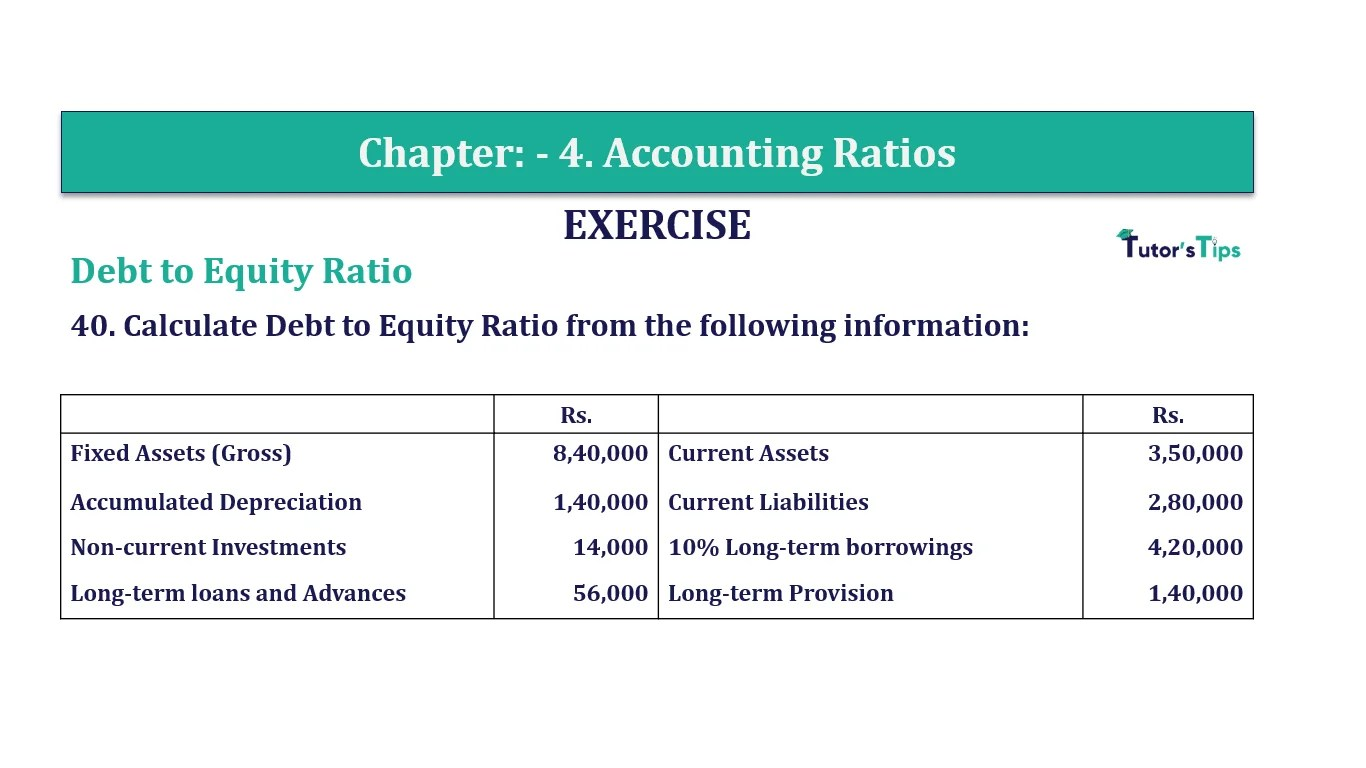# Question 40 Chapter 4 of +2-B – T.S. Grewal 12 ClassQuestion No. 40- Chapter No.4 - T.S. Grewal +2 Book Part B

Question 40 Chapter 4 of +2-B

Debt to Equity Ratio

40. Calculate Debt to Equity Ratio from the following information:

 Rs. Rs. Fixed Assets (Gross) 8,40,000 Current Assets 3,50,000 Accumulated Depreciation 1,40,000 Current Liabilities 2,80,000 Non-current Investments 14,000 10% Long-term borrowings 4,20,000 Long-term loans and Advances 56,000 Long-term Provision 1,40,000

Calculate ratios indicating the Long-term and the Short-term financial position of the company.

### The solution of Question 40 Chapter 4 of +2-B: –

 Equity = Total Assets – Total Debts = (8,40,000 – 1,40,000) + 14,000 + 56,000 + 3,50,000 – (4,20,000 + 1,40,000 + 2,80,000) Equity = Rs. 2,80,000 Debt = Long-Term Borrowings + Long-Term Provisions = Rs. 4,20,000 + Rs. 1,40,000 Debt = Rs. 5,60,000

 Debt to Equity Ratio = Debts = Rs.5,60,000 Equity Rs.2,80,000 = 2: 1

Balance Sheet: Meaning, Format & Examples

Comment if you have any question.

Also, Check out the solved question of previous Chapters: –